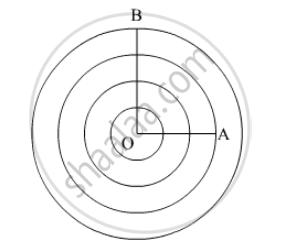# The Radius of a Circle is 20 Cm. It is Divided into Four Parts of Equal Area by Drawing Three Concentric Circles Inside It. Then, the Radius of the Largest of Three Concentric Circles Drawn is - Mathematics

MCQ

The radius of a circle is 20 cm. It is divided into four parts of equal area by drawing three concentric circles inside it. Then, the radius of the largest of three concentric circles drawn is

#### Options

• 10 $\sqrt{5}$

• 10$\sqrt{3}$cm

• 10$\sqrt{5}$

•  10$\sqrt{2}$

#### Solution

The circle can be divided into four parts of equal area by drawing three concentric circles inside it as,It is given that OB = 20 cm. Let OA = x.

Since the circle is divided into four parts of equal area by the three concentric circles, we have,

Area of the fourth region =1/4xxArea of the given circle

pixx(20^2-x^2)=1/4xxpixx20^2

400-x^2=100

x^2=300

x-10sqrt3 cm

Concept: Area of Circle
Is there an error in this question or solution?
Chapter 13: Areas Related to Circles - Exercise 13.6 [Page 73]

#### APPEARS IN

RD Sharma Class 10 Maths
Chapter 13 Areas Related to Circles
Exercise 13.6 | Q 40 | Page 73

Share